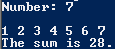# Assignment #88 and Adding Values with a For Loop

## Code

```    /// Name: Ali Kurland
/// Period: 6
/// Program Name: Adding Values with a For Loop
/// Date Finished: 10/28/15

import java.util.Scanner;

{
public static void main( String[] args )
{
Scanner keyboard = new Scanner(System.in);

int number, sum = 0;

System.out.print( "Number: " );
number = keyboard.nextInt();

System.out.println( " " );

for ( int n = 1 ; n <= number ; n = n+1 )
{
System.out.print( n + " " );
sum = sum + n ;
}

System.out.println( " " );
System.out.println( "The sum is " + sum + "." );
}
}
```

### Picture of the output